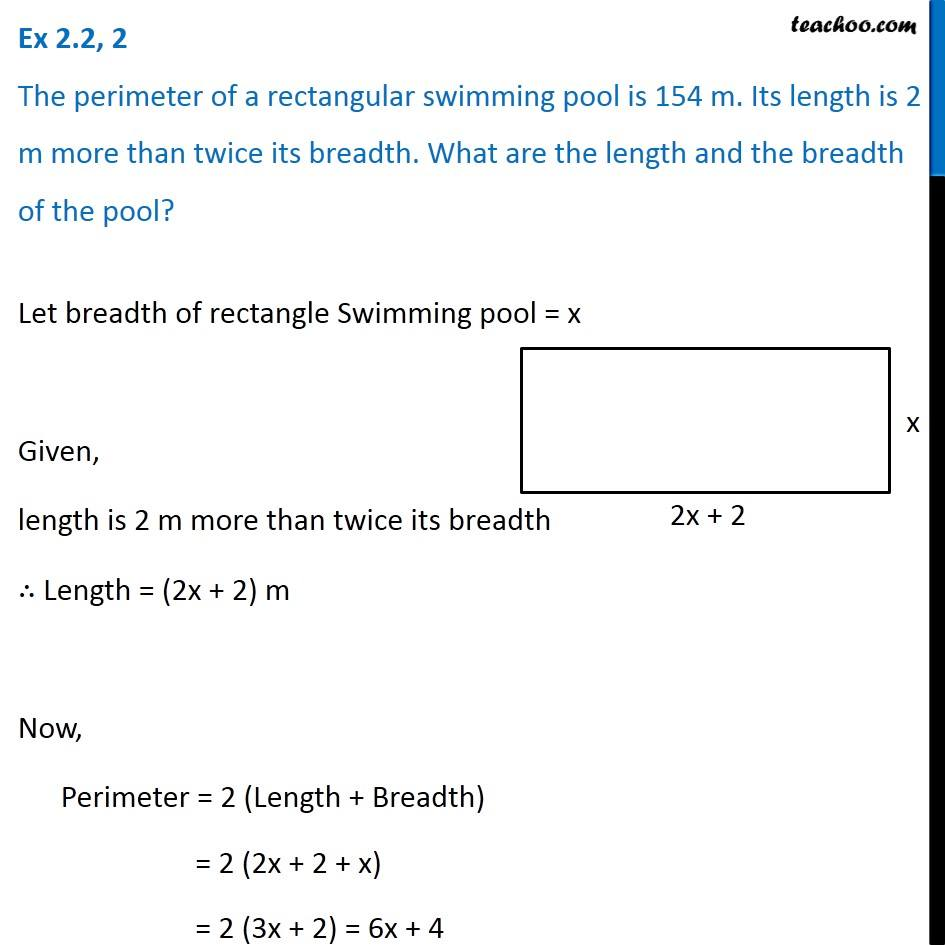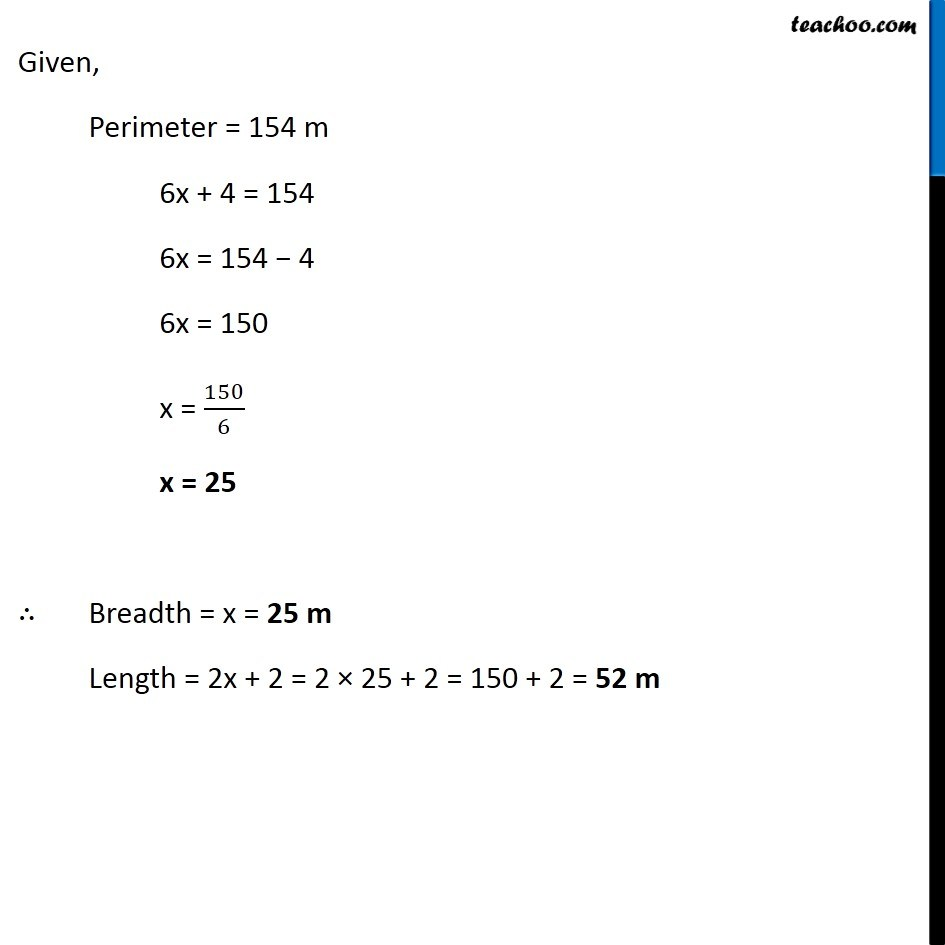Ex 2.2

Chapter 2 Class 8 Linear Equations in One Variable
Serial order wiseGet live Maths 1-on-1 Classs - Class 6 to 12

### Transcript

Ex 2.2, 2 The perimeter of a rectangular swimming pool is 154 m. Its length is 2 m more than twice its breadth. What are the length and the breadth of the pool?Let breadth of rectangle Swimming pool = x Given, length is 2 m more than twice its breadth ∴ Length = (2x + 2) m Now, Perimeter = 2 (Length + Breadth) = 2 (2x + 2 + x) = 2 (3x + 2) = 6x + 4 Given, Perimeter = 154 m 6x + 4 = 154 6x = 154 − 4 6x = 150 x = 150/6 x = 25 ∴ Breadth = x = 25 m Length = 2x + 2 = 2 × 25 + 2 = 150 + 2 = 52 m